Most Affordable JEE | NEET | 8,9,10 Preparation by Kota's Top IITian Doctor Faculties

# Isomerism - JEE Advanced Previous Year Questions with Solutions`
JEE Advanced Previous Year Questions of Chemistry with Solutions are available at eSaral. Practicing JEE Advanced Previous Year Papers Questions of Chemistry will help the JEE aspirants in realizing the question pattern as well as help in analyzing weak & strong areas. Simulator Previous Years JEE Advance Questions
Q. The correct statement(s) about the compound $\mathrm{H}_{3} \mathrm{C}$ (HO)HC – CH = CH – CH(OH) $\mathrm{CH}_{3}$ (X) is (are) : (A) The total number of stereoisomers possible for X is 6 (B) The total number of diastereomers possible for X is 3 (C) If the stereochemistry about the double bond in X is trans, the number of enantiomers possible for X is 4 (D) If the stereochemistry about the double bond in X is cis, the number of enantiomers possible for X is 2 [iit-2009]
Ans. (A,D)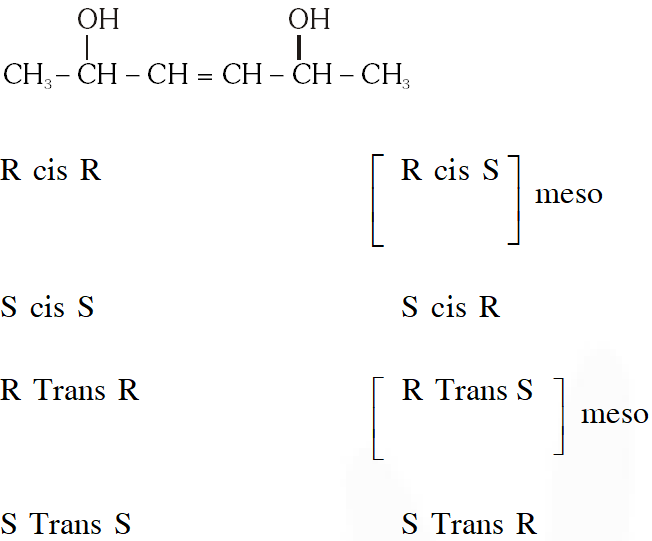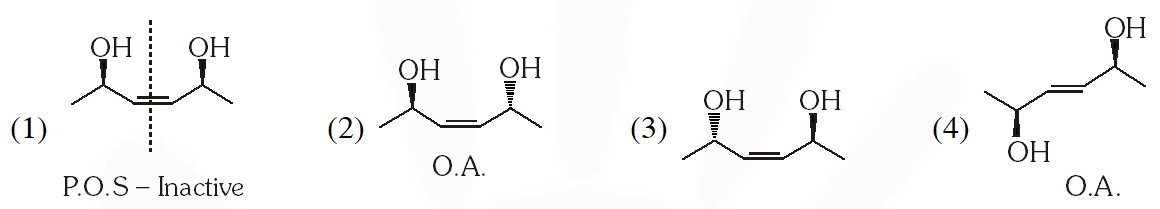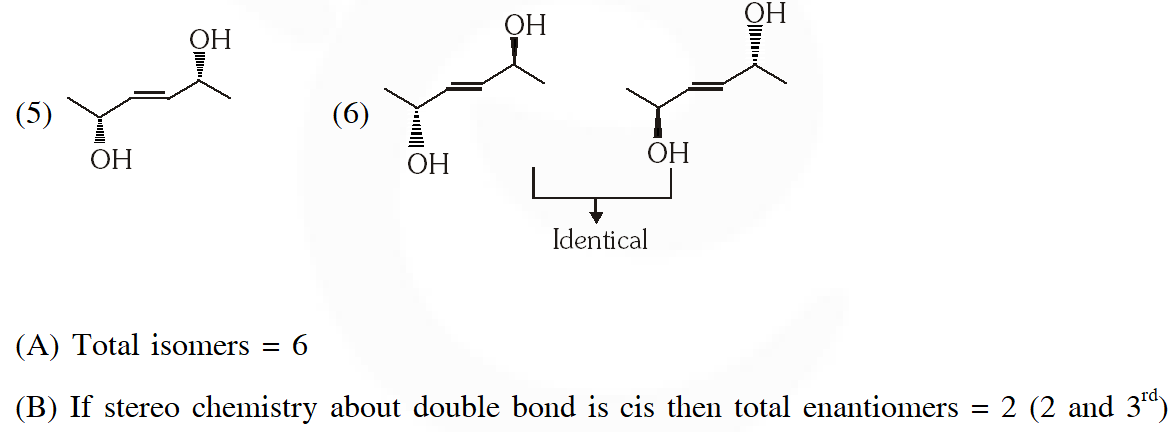Q. In the Newman projection for 2,2–dimethylbutane –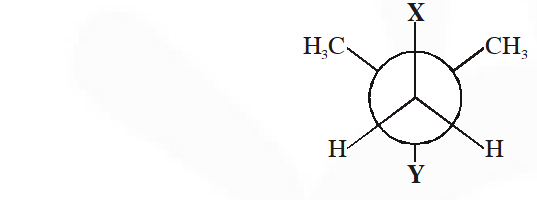X and Y can respectively be – (A) H and H (B) $\mathrm{H}$ and $\mathrm{C}_{2} \mathrm{H}_{5}$ (C) $\mathrm{C}_{2} \mathrm{H}_{5}$ and $\mathrm{H}$ (D) $\mathrm{CH}_{3}$ and $\mathrm{CH}_{3}$ [iit-2010]
Ans. (B,D)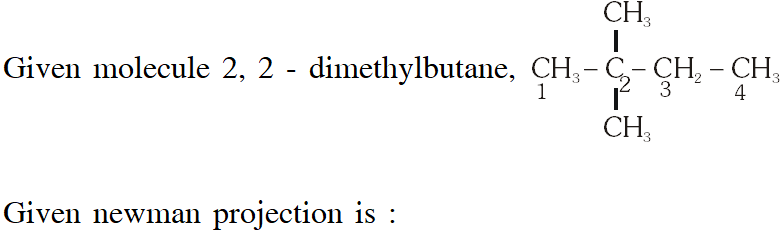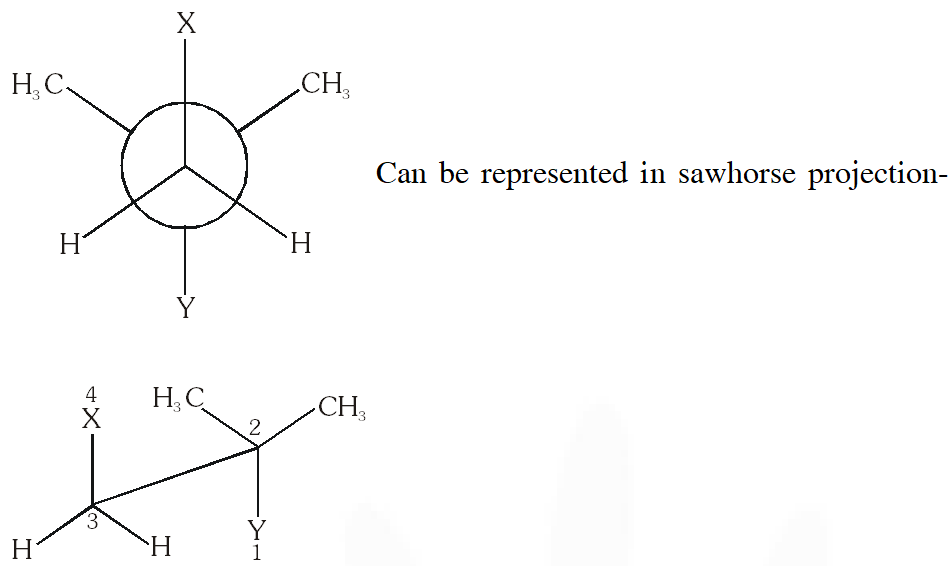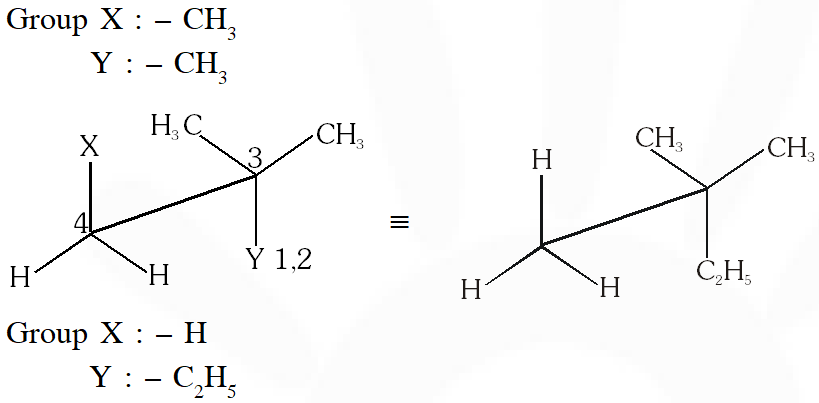Q. Amongst the given option, the compound(s) in which all the atoms are in one plane in all the possible conformations (if any), is (are) –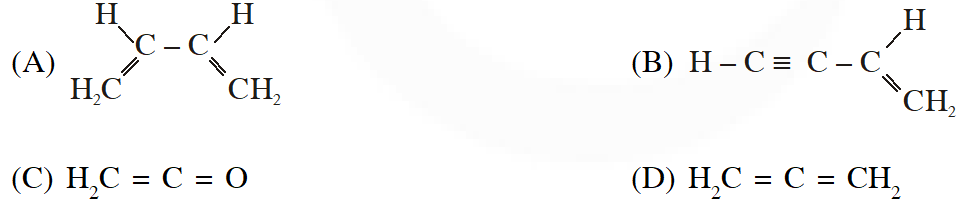[iit-2011]
Ans. (B,C)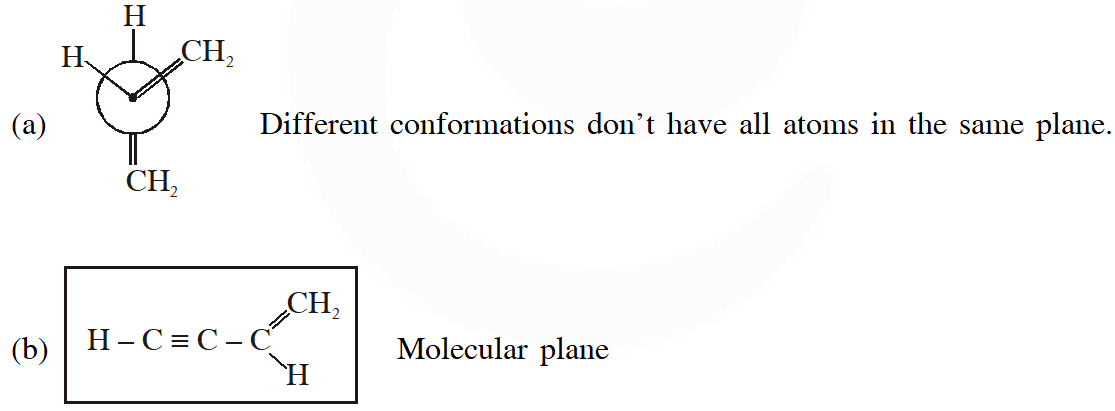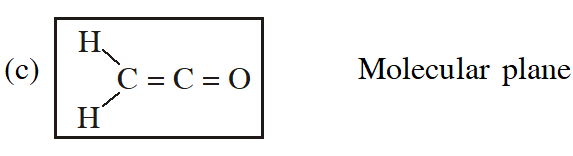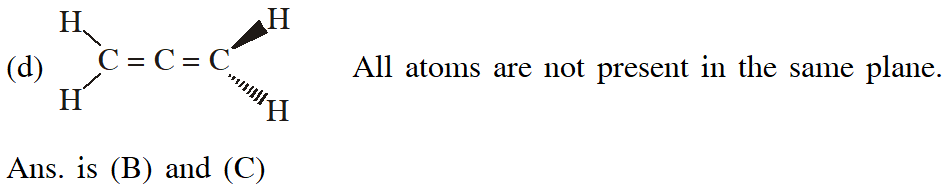Q. Which of the given statement(s) about N,O,P and Q with respect to M is (are) correct ?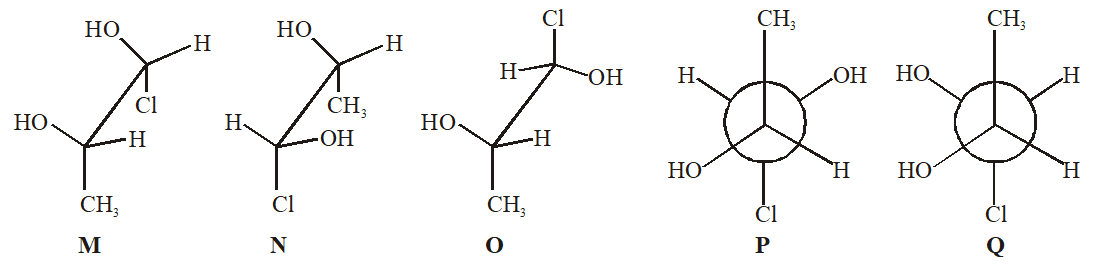(A) M and N are non-mirror image stereoisomers (B) M and O are identical (C) M and P are enantiomers (D) M and Q are identical [JEE-2012]
Ans. (A,B,C)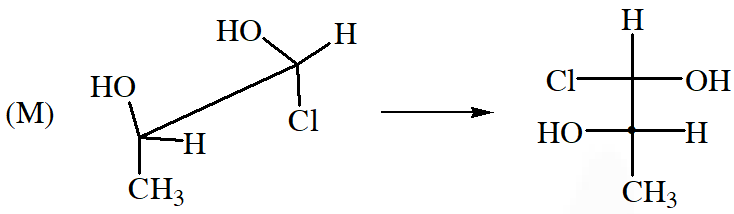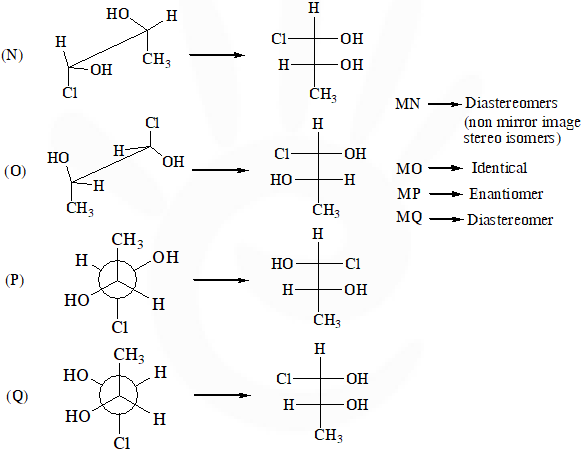Q. The total number(s) of stable conformers with non-zero dipole moment for the following compound is (are) :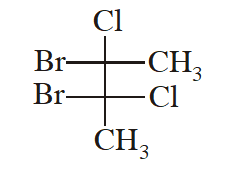[JEE-2014]
Ans. 3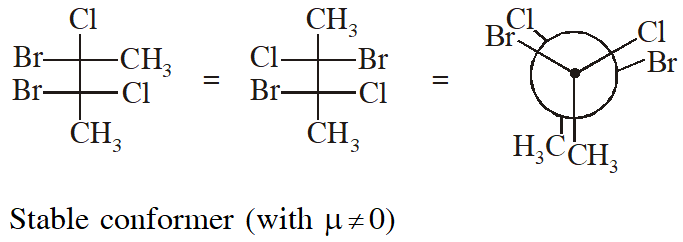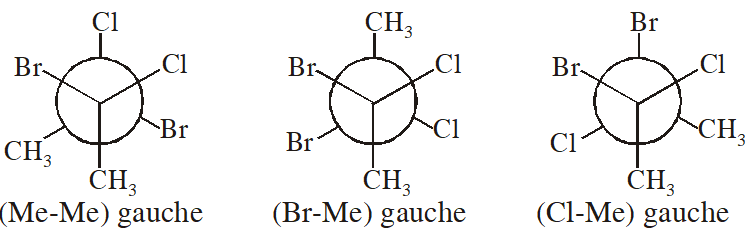April 14, 2023, 6:35 a.m.
Excellent
April 14, 2023, 6:35 a.m.
Excellent
Surya
Sept. 11, 2022, 10:30 p.m.
2015 se 2022 Tak dalo
Krish
Aug. 6, 2022, 10:04 p.m.
Fantastic
🖕🖕🖕
July 6, 2022, 4:57 p.m.
Saalo ne 15se 21 Tak ke nahi daale questions
Tharo baap
Nov. 19, 2021, 9:22 a.m.
Fake reviews
niyat
May 28, 2021, 9:56 p.m.
life is monotonous
Jan. 31, 2021, 7:35 p.m.
Very nice
D
Oct. 4, 2020, 8:51 p.m.
What is this